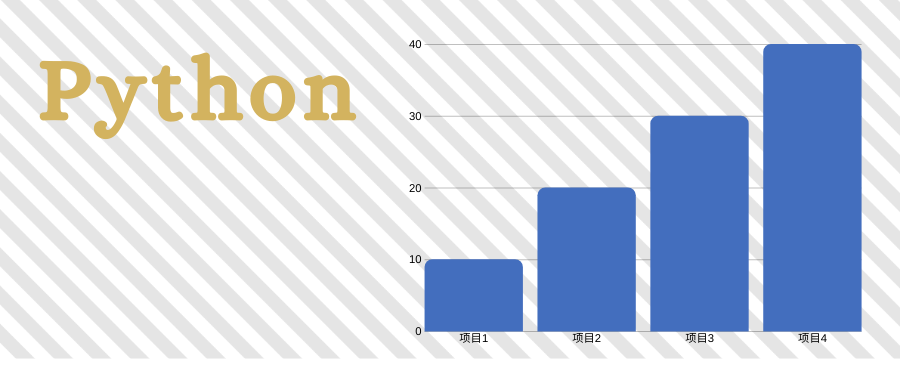# Python 中怎样合并数据2020 年 05 月 07 日 阅读数: 3# 2. 分析

## 2.1. list

list 的连接是最容易的，直接用 Python 语法即可。

a = [1, 2, 3]b = [4, 5, 6]c = a + bprint(c)d = [a, b]print(d)

[1, 2, 3, 4, 5, 6][[1, 2, 3], [4, 5, 6]]

## 2.2. dict

dict 不支持 +。想要合并两个dict，需要用到**操作符。

a = {'a': 1, 'b':2, 'c': 3}b = {'d': 4, 'e':5, 'f': 6}c = {**a, **b}print(c)

{'a': 1, 'b': 2, 'c': 3, 'd': 4, 'e': 5, 'f': 6}

a = {'a': 1, 'b':2, 'c': 3}b = {'a': 4, 'e':5, 'f': 6}c = {**a, **b}print(c)

{'a': 4, 'b': 2, 'c': 3, 'e': 5, 'f': 6}

## 2.3. set

set 不可 hashable，而且不支持+，所以需要另想办法。

a = set([1, 2, 3])b = set([1, 5, 6])c = a.union(b)print(c)d = {item for item in list(a) + list(b)}print(d)

{1, 2, 3, 5, 6}{1, 2, 3, 5, 6}

## 2.4. ndarray

ndarray 合并的时候，需要注意坐标轴方向和相应方向大小是否吻合。比如

import numpy as npa = np.array([[1, 2, 3], [4, 5, 6]])b = np.array([[7, 8, 9], [10, 11, 12]])c = np.concatenate((a, b))print(c)d = np.concatenate((a, b), axis=1)print(d)

[[ 1  2  3] [ 4  5  6] [ 7  8  9] [10 11 12]][[ 1  2  3  7  8  9] [ 4  5  6 10 11 12]]

a = np.array([[1, 2, 3], [4, 5, 6]])b = np.array([[7, 8, 9]])c = np.concatenate((a, b))print(c)d = np.array([[7, 8]]).transpose()e = np.concatenate((a, d), axis=1)print(e)

[[1 2 3] [4 5 6] [7 8 9]][[1 2 3 7] [4 5 6 8]]

a = np.array([[1, 2, 3], [4, 5, 6]])b = np.array([7, 8])c = b[:, np.newaxis]d = np.concatenate((a, c), axis=1)print(d)

array([[1, 2, 3, 7],       [4, 5, 6, 8]])

## 2.5. DataFrame

DataFrame 比较灵活，可以在各个维度上进行扩展。所以连接的时候需要特别注意方向。

import pandas as pda = pd.DataFrame([[1, 2, 3], [4, 5, 6]], columns=['a', 'b', 'c'])b = pd.DataFrame([[7, 8], [9, 10]], columns=['b', 'd'])c = pd.concat([a, b], axis=0)cd = pd.concat([a, b], axis=1)d

	a	b	c	d0	1.0	2	3.0	NaN1	4.0	5	6.0	NaN0	NaN	7	NaN	8.01	NaN	9	NaN	10.0	a	b	c	b	d0	1	2	3	7	81	4	5	6	9	10

# 4. 交流Lily

# 5. 扩展

## 5.1. 延伸阅读

1. Numpy 中如何对矩阵的特征对排序

## 5.2. 参考文献

1. M. Wes, Python for Data Analysis, 2nd. Beijing: O’Reilly, 2017.

2. J. VanderPlas, Python Data Science Handbook. Beijing: O’Reilly, 2016.Data2Science## 评论